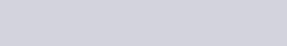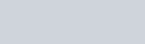# Correlation Analysis definition, formula and step by step procedure

The relationship between two or more random variables are generally defined as the correlation. It is the major part of bivariate analysis. When variables are found to be related, we often want to know how close the relationship is. The study of the relationship is known as correlation analysis. The primary objective of correlation is to measure the strength or degree of linear association between two or more variables.

## Example Correlation Analysis

For example, we may be interested in measuring the relationship between the-

• Height and weight of the people of certain area.
• Ages of husband and their wives.
• Amount of rice production and fertilizer.
• Income and expenditure.
• Total sales and experience of the sales persons.. etc.

### Correlation analysis vs Regression analysis

The contradictions between regression and correlation are given below-

• In correlation, we are generally interested in the measurement of the linear relationship between two or more variables. On the other hand, regression analysis doesn’t asses such relationship.
• In correlation analysis we consider any two or more variables. On the other hand, in regression there must need one dependent and one or more independent variables. Here the dependent variable is stochastic or random variable and the independent or explanatory variable is fixed.
• Correlation analysis provides a means of measuring the goodness of fit of the estimated regression line to the observed statistical data. On the other hand, regression analysis doesn’t provide any means to measure the goodness of fit but it tells about the average amount of change in the dependent variable to one unit change in the independent variable.

### Measuring the Correlation

For n pairs of sample observations (x1,y1), (x2,y2),…,( xn, yn), the correlation coefficient  r can be defined as,Correlation coefficient r is a statistical measure that quantifies the linear relationship between a pair of variables.

### Interpretation of Correlation Coefficient

The value of correlation coefficient (r) lies between -1 to +1. When the value of –

• r=0; there is no relation between the variable.
• r=+1; perfectly positively correlated.
• r=-1; perfectly negatively correlated.
• r= 0 to 0.30; negligible correlation.
• r=0.30 to 0.50; moderate correlation.
• r=0.50 to 1 highly correlated.

### Properties of correlation coefficient

The correlation coefficient has some appealing properties which are following-

• The correlation coefficient is a symmetric measure.
• The value of correlation coefficient lies between -1 to +1.
• It is dimensionless quantity.
• It is independent of origin and scale of measurement.
• The correlation coefficient will be positive or negative depending on whether the sign of numerator of the formula is negative or positive.

### Rank Correlation analysis

When the two variables had a joint normal distribution and the conditional variance of one variable given the other was same then we may use other technique generally known as the rank correlation. Rank correlation is defined by Spearman’s rank correlation. We recommended rank correlation when-

• The values of the variables are available in rank ordered form.
• The data are qualitative in nature and can be ranked in some order.
To compute Spearman’s rank correlation  we use the following formula-where,

rs=Spearman’s correlation coefficient

di=The differences between ranks of the ith pair

n= The number of pairs included.

Try some fresh content: